## Tuesday, May 30, 2017

### How To Derive the Prime Number Theorem

I read the article on Wikipedia about prime number distribution and I was surprised to find that the inverse logarithimc function was a very recent (late-nineteenth century) innovation, and that the proof was very deep. I have my own derivation which seems fairly simple, and I wonder if it is correct; and if it is, whether it has been previously written up.

It’s based on the Sieve of Eratosthenes, and it goes like this. You know that when checking for primes, you only have to go up to the square root of the number you’re testing. So if you’re looking for primes in the vicinity of 1,000,000 you only have to work the seive up to 1000.

Now, let’s say you’re checking for primes in the vicinity of 1,200,000. Now you have to work the sieve a little farther – in fact, up to about 1100. So the sieving process is going to be more thorough. That’s why the density of primes goes down as you move upwards.

How much more thorough is the sieve going to be? It will be more thorough by exactly the number of primes between 1000 and 1100. In short, the rate of change of the density function in the vicinity of 1000000 depends on the value of the density function in the vicinity of 1000. Can we construct a differential equation from these constraints?

Yes we can, and you will see that when we solve it we get 1/lnx, the desired result which eluded mathematicians for so long. Yes, there are more accurate functions for the distribution, but this is ground zero, the starting point.

- - - - - - - - -

Would you like to see how I construct the differential equation? You might not like how I do it, but it works for me. I find it’s not so hard if you throw some numbers into the game. Let’s suppose that the density of primes in the vicinity of 1000 is 1/8. So there are approximately 12.5 primes between 1000 and 1100. (The numbers don’t matter…we’re going to throw them away in the end and convert them into functions, variables, and differentials. But I like having numbers in front of me.)

Now let’s assume that the density of primes in the vicinity of 1 million is 1/40. So there are 25 primes between 1,000,000 and 1.001, 000? Then we ask: how many primes are there between 1,2000,000 and 1,201, 000

Well, if you applied the same Sieve of Eratosthenes to the higher interval, you would get the same number of primes – 25 primes. But in fact…the Sieve of Eratosthenes is more severe on the higher interval. You have an additional 12.5 primes between 1000 and 1100, and each of them strikes the new interval exactly once. (I’ve sized the interval so it works out that way.)

Let us call a number a prime candidate in the new interval if it survived the Sieve up to 1000. There are of course 25 prime candidates. How many will be struck by the sieve over the interval 1000 to 1100?

At first glance you might think we will lose half (12.5) of the prime candidates. But of course it’s not so. Most of the new sieving numbers fall on those 975 composite numbers that have already been eliminated. A little careful thought shows that if the sieving is truly random, the chance that a given number will be struck by the additional impact is 12.5/1000. So of the 25 prime candidates, we stand to lose only .375, leaving 24.625 prime numbers in the interval from 1.200 million to 1.201 million.

Shall we now convert the preceding narrative into a differential equation? It’s not so hard. Let’s consider n as being 1000 and dn = 100.  then  (using Greek rho for density) ρ(n) = 1/8.

Now let’s look at the density in the neighborhood of 1,000,000…that is,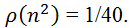We’re going to ask how fast ρ is changing as you go from 1 million to 1.2 million…in other words from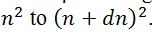.

We’ve already done this with numbers. The number of primes between 1 million and 1.001 million is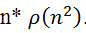The number of primes between 1.2 million and 1.201 million is, formally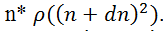But we’ve already figured out how to calculate this. It’s just the same as the number in the vicinity of 1 million, subtract a correction which we’ve figured out as: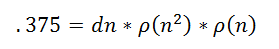We are very close to having our differential equation. The density of prime numbers in the vicintiy of 1.2 million is just (25 - .375)/1000, or converting this all into symbols: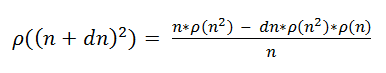This is a differential equation and it solves. Multiplying and regrouping, we get: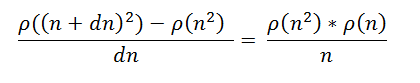From here we get: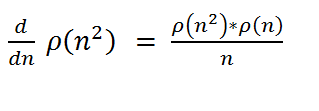I’m not gonna pretend I can solve this diffrerential equation, but I know how to substitute in. And if I put 1/ln(n) in for the density, I get a true equation. Try it yourself if you don’t believe me.